Test Prep Plan - Take a practice test

Take this practice test to check your existing knowledge of the course material. We'll review your answers and create a Test Prep Plan for you based on your results.
How Test Prep Plans work
1
2Based on your results, we'll create a customized Test Prep Plan just for you!
3Study smarter
Study more effectively: skip concepts you already know and focus on what you still need to learn.

Algebra II: Complex and Imaginary Numbers Chapter Exam

Exam Instructions:

Choose your answers to the questions and click 'Next' to see the next set of questions. You can skip questions if you would like and come back to them later with the "Go To First Skipped Question" button. When you have completed the practice exam, a green submit button will appear. Click it to see your results. Good luck!

Page 1

Question 1 1. Simplify the following expression: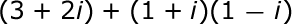Question 2 2. Simplify the following expression.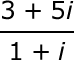Question 3 3. How many roots does the equation below have?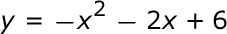Question 4 4. Which statement MUST be true about the equation below?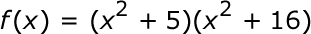Question 5 5. Which number is represented by the graph below?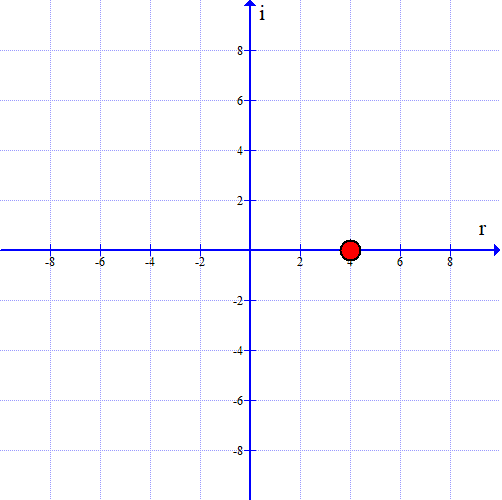Page 2

Question 6 6. Simplify the following expression: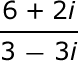Question 7 7. What is the simplified value of the expression below?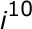Question 8 8. Add and simplify the following expression: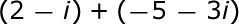Question 10 10. Multiply and simplify the following expression: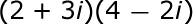Page 3

Question 13 13. What is the first step in dividing these complex numbers?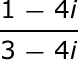Question 15 15. Solve the equation below: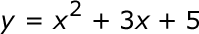Page 4

Question 16 16. Subtract and simplify the following expression: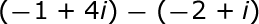Question 17 17. Find the roots of the equation below: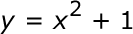Question 18 18. What is the most simplified form of the expression below?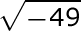Question 19 19. Which number is represented by the graph below?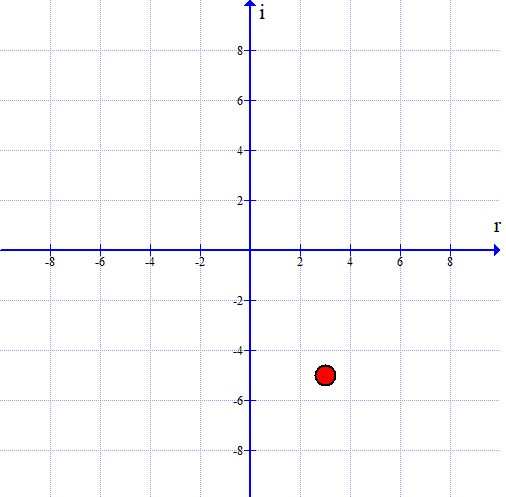Page 5

Question 21 21. What is the complex conjugate of the expression below?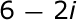Question 22 22. What type of solution(s) would you get from the equation below?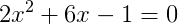Algebra II: Complex and Imaginary Numbers Chapter Exam Instructions

Choose your answers to the questions and click 'Next' to see the next set of questions. You can skip questions if you would like and come back to them later with the "Go To First Skipped Question" button. When you have completed the practice exam, a green submit button will appear. Click it to see your results. Good luck!

Support